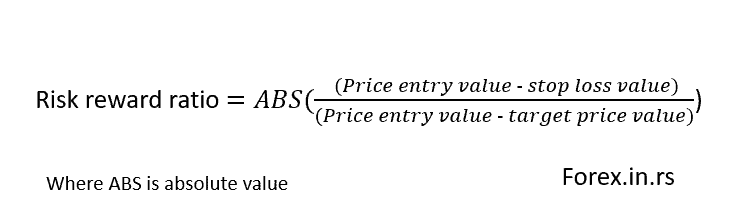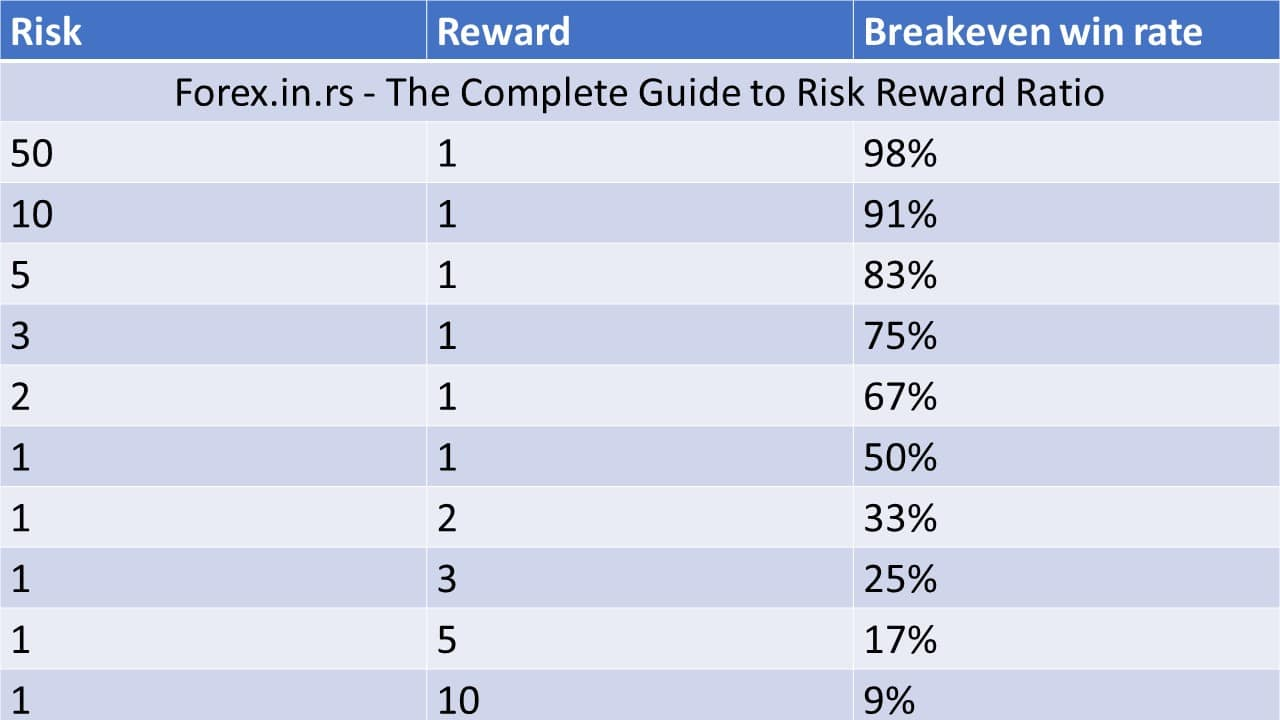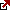# How to Calculate Risk Reward Ratio in Forex?

Trading is a fascinating and rewarding endeavor that has captured the imagination of many individuals worldwide. From amateur investors to seasoned professionals, the allure of making fortunes from buying and selling stocks, currencies, and commodities is simply irresistible. While many factors contribute to a trader’s success or failure, one of the most critical ones is the concept of “position risk” and “position reward.”

At its core, position risk refers to the possibility of sustaining losses due to holding a particular investment or position. But, it is the amount of money an investor will lose if the market moves against them. On the other hand, position reward is the potential profit that can be made from a particular investment or position. It is the amount of money that an investor stands to gain if the market moves in their favor.

The simple risk-reward definition we gave in our article Risk-Return Ratio – Risk Reward Ratio Explained:

## What is the Risk reward ratio?

The risk-reward ratio or risk-return ratio in trading represents a given trade’s expected return and risk based on the entry and close positions. A good risk-reward ratio tends to be less than 1 when the return (reward) is greater than the risk.

If you need to use a risk-reward calculator try an excellent tool.

The risk-reward ratio measures the reward level you could achieve when you complete a trade-in correlation to each dollar you are willing to put up to risk. Consider, for example, that if the risk-reward ratio is shown to be 1:3, you are willing to put up one dollar to risk to gain three dollars in reward. Or if the risk-reward ratio is set forth as 1:5, this denotes that you are willing to put one dollar up to risk to make a profit of five dollars.

A good risk-reward ratio means the potential reward is higher than the potential risk, while a bad risk-reward ratio indicates that the potential risk outweighs the potential reward.

Here are examples of both scenarios:

Good Risk-Reward Ratio: Example: A trader enters a trade with a risk-reward ratio of 1:2. They set a stop-loss order at 50 pips below the entry price and a take-profit order at 100 pips above the entry price. If the trade goes in their favor, they will gain twice the amount they risked.

In this scenario, if the trade is successful, the trader will earn a reward twice the size of the risk they took. Even if they have a few losing trades, they can still be profitable in the long run by maintaining a consistently favorable risk-reward ratio.

Bad Risk-Reward Ratio: Example: A trader enters a trade with a risk-reward ratio of 2:1. They set a stop-loss order at 100 pips below the entry price and a take-profit order at only 50 pips above the entry price.

In this case, the trader risks twice as much as their potential reward. Therefore, if the trade goes against them, they will incur a loss twice the size of their potential gain. This unfavorable risk-reward ratio makes it challenging for the trader to achieve consistent profitability, as they would need a higher win rate to compensate for the limited reward compared to the risk.

## How to calculate the risk-reward ratio in forex?

To calculate the risk-reward ratio in forex, you need to divide the difference between the entry point price and stop-loss price level (risk) by the difference between the profit target and the entry point price level (reward).

If the risk is greater than the reward (for example, 4:1), the ratio is greater than 1; if the reward is greater than the risk (for example, 1:3), the ratio is less than 1.

## Risk-reward ratio formula

Risk-reward ratio = absolute value (Price entry value – stop loss value) / absolute value (Price entry value – target price value)The risk-reward ratio formula is a simple calculation used to determine the potential reward relative to the potential risk in a trade. It is calculated by dividing the absolute difference between the entry and stop loss prices by the difference between the entry and target prices.

## Steps to calculate risk-reward ratio

1. Identify the entry price: The entry price is when a trader enters a trade. For example, it could be the current market price or a specific price level at which the trader initiates the trade.
2. Determine the stop loss price: The stop loss price is the predetermined price level at which a trader will exit the trade to limit their potential losses. It is set based on the trader’s risk tolerance and analysis of the market conditions.
3. Determine the target price: The target price is the desired price level at which a trader expects to profit and exit the trade. It is typically based on the trader’s analysis of the market and their profit targets.
4. Calculate the absolute difference between the entry price and the stop loss price: Subtract the stop loss price from the entry price, and take the absolute value of the result. This ensures that the value is always positive, regardless of whether the stop loss price is higher or lower than the entry price.
5. Calculate the absolute difference between the entry and target prices: Subtract the target price from the entry price, and take the absolute value of the result.
6. Divide the absolute difference between the entry and stop loss prices by the difference between the entry and target prices: This division gives the risk-reward ratio. The resulting ratio indicates how often the potential reward exceeds the potential risk.

The risk-reward ratio provides traders with a quantitative measure of the potential profitability of trading relative to the potential loss. A favorable risk-reward ratio, such as 1:2 or higher, means that the potential reward is at least double the potential risk. Traders often seek higher risk-reward ratios to ensure that their winning trades outweigh their losses in the long run.

For example, based on this risk-reward formula, if we buy EURUSD and the entry price is 1.3, and stop loss is 1.2, and the target is 1.5, then:

(Entry price – stop-loss) = 100 pips (from 1.3 till 1.2, there are 100 pips.)
(Entry price – target price) = 200 pips (from 1.3 till 1.5, there are 200 pips.)

Risk reward ratio = 100/200= 1/2. Risk is one and reward 2, 1:2.

This is the best way how to calculate the risk-reward ratio in trading.

## Risk reward ratio myth

I listen to all the time expressions – High-risk, high reward!

The risk-return spectrum says that the risk-reward is the relationship between the amount of return gained on an investment and the amount of risk undertaken in that investment. This rule exists in all kinds of business, not just forex. Many traders are seeking low-risk trades where they try to risk a few pips and achieve high returns. The problem with this approach is the shallow winning rate. Psihologicly, this can be very stressful for traders.

The risk-reward ratio may fool some people who do not really know what it is or do not understand it well. If you are used to seeking trades with a risk-reward ratio of 1:2, you could likely continue to be the loser every time.

It is realized that when a trader seeks trades that tend to possess a risk-reward ratio lower than 1, the trader can potentially continue to be profitable consistently. Then this leads one to ask why this is so. This is because the risk-reward ratio is only one factor relating to the success achieved.

Now in terms of the lie that you have been given regarding the risk-reward ratio, you likely have been told that you must possess a risk-reward ratio set at 1:2. But that is not true. This is based on the premise that the risk-reward ratio does not carry much merit. Consider, for example, that the risk-reward ratio has been set at 1:2. This indicates that every trade you are perceived as winning will yield you two dollars in profits. However, the fact is that your winning rate may only be twenty percent. This means that you may win only two trades when you have engaged in ten trades, losing the remaining eight trades.

As a result, when the math is completed, this denotes that your total loss is eight dollars, and your total gain is four dollars. Thus, it is determined that your net loss is four dollars.

With this being the case, it is highly evident that it is imperative to comprehend that applying the risk-reward ratio usage by itself as a metric is fruitless. Instead, combining the risk-reward ratio with your winning rate is necessary to determine if you will make profits in the long term–which is also referred to as your expectancy.

## How to use the risk-reward ratio to be profitable?

Risk reward ratio traders must use the Winning and Kelly ratios to create better position size and improve trading performance. For example, if you use a 1:5 risk-reward ratio and your winning ratio is 3%, it isn’t good, even if you have an excellent risk-reward ratio.You need to know the secret to be profitable. Thus, you must divide the size of your winning by the size of your average loss and add one. Then you are to multiply this by your winning rate and subtract one. This will determine your expectancy when you are conducting trades.

In a scenario where you conduct ten trades, six are counted as winning trades. On the other hand, four are counted as losing trades. Therefore, the result is that your winning percentage ratio is expressed as sixty percent or as six over ten. If the case is that six winning trades equated to granting you profits for three thousand dollars, it is commonly understood that your average win is regarded as five hundred dollars. This is derived by dividing the number of profits of three thousand dollars by the number of winning trades, which were determined to be six wins.

We wrote two articles on this website: Money management expert advisor and How do you Profit from Forex Trading? In both articles, we are talking about the Kelly ratio.

Position size = Winrate – ( 1- Winrate / Risk reward Ratio)

In such a case that four of the trades were losses that equated to one thousand and six hundred dollars, your average loss is determined to be four hundred dollars. This was derived by dividing the total money lost by the number of your losing trades.

Now it is time to apply this to the previously addressed formula. Thus, you will divide your winning trade average by the losing trade average and add one to this amount. Then you will multiply that by the percentage of your winning trades and minus one from the amount. Thus, the result is that your determined expectancy is considered to be 0.35, or it may be expressed as thirty-five percent. This is regarded as being a relatively positive expectancy. This means you will likely receive thirty-five cents for every dollar you trade over the long term.

Thus, it is realized that the truth is that there is no foundational minimum risk-reward ratio of 1:2. This is because one may possess a risk-reward ratio set at 1:0.5; yet, if the person possesses a high enough winning rate, then the trader will still gain profits over the long term.

With this being the case, the primary metric for consideration in terms of importance is not to be viewed as the risk-reward ratio. Further, it is not to be viewed as being your winning rate. Instead, the most crucial metric factor to consider at this point is your expectancy rate.

With this perspective in place, it is realized that it is not a good idea to engage in the placement of a stop loss at a rate that is considered to be arbitrary, such as one hundred pips up to three hundred pips, as this effort would not result in making much sense. But instead, it is believed that it is best to engage in leaning against the factors of the markets that serve as barriers, as they engage in the prevention of the price point from aiming at landing on your stops.

Day Trading with Risk/Reward Ratios and Win Rate

So, what is an excellent risk-reward ratio? The good risk-reward ratio is the ratio that gives the best profit with a combined winning rate. So alone, without other rates, the risk-reward ratio is not an important parameter.

If your trading strategy offers you more trades, then it can be sensible to consider that you are losing profits. By the day’s end, winning trades may not always end up with profits,

The day trades should assess the worth of losses and wins based on their risk-reward ratio, win-loss ratio, acceptable risks, and losses while creating an ask or bid.

To be a successful day trader, you can generate equilibrium between the risk-reward and win-rate ratios by considering all the elements. For example, your risk-reward ratio should be 1.0 if the win rate is higher, like 60-70%, and for a win rate of 40-50%, it should be around 0.69-0.65.

Fxigor

Igor has been a trader since 2007. Currently, Igor works for several prop trading companies. He is an expert in financial niche, long-term trading, and weekly technical levels. The primary field of Igor's research is the application of machine learning in algorithmic trading. Education: Computer Engineering and Ph.D. in machine learning. Igor regularly publishes trading-related videos on the Fxigor Youtube channel. To contact Igor write on: igor@forex.in.rs

Trade gold and silver. Visit the broker's page and start trading high liquidity spot metals - the most traded instruments in the world.